# How to calculate compound interest in Excel - formula for daily, monthly, yearly compounding

The tutorial explains the compound interest formula for Excel and provides examples of how to calculate the future value of the investment at annual, monthly or daily compounding interest rate. You will also find the detailed steps to create your own Excel compound interest calculator.

Compound interest is one of the basic building blocks in banking and one of the most powerful financial forces around that determine the outcome of your investments.

Unless you are an accounting graduate, financial analyst or an experienced investor, it might be a bit difficult to grasp the concept from specialized financial books and manuals. The aim of this article is to make it easy : ) You will also learn how to use a compound interest formula in Excel and create a universal compound interest calculator for your own worksheets.

## What is compound interest?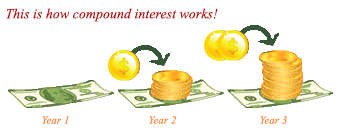In very simple terms, compound interest is the interest earned on interest. More precisely, compound interest is earned on both the initial deposit (principal) and the interest accumulated from previous periods.

Perhaps, it might be easier to start with simple interest that is calculated only on the principal amount. For example, you put \$10 into a bank account. How much will your deposit be worth after one year at an annual interest rate of 7%? The answer is \$10.70 (10 + 10*0.07 = 10.70), and your earned interest is \$0.70.

In case of compound interest, the principal in each time period is different. The bank won't give the earned interest back to you, instead they add it to your principal investment. This increased amount becomes the principal for the next time period (compounding period) and also earns interest. In other words, you earn interest not only on the principal amount, but also on the interest earned in each compounding period.

In our example, in addition to the principal amount of \$10, the earned interest of \$0.70 will also earn interest next year. So, how much will your \$10 deposit be worth after 2 years at the annual interest rate of 7% compounded yearly? The answer is \$11.45 (10.7 + 10.7*0.07 = 11.45) and your earned interest is \$1.45. As you see, at the end of the second year, not only did you earn \$0.70 on the initial \$10 deposit, you also earned \$0.05 on the \$0.70 interest that accumulated in the first year.

There are several ways to calculate compound interest in Excel, and we are going to discuss each one in detail.

## How to calculate compound interest in Excel

Long time investments can be an effective strategy to increase your wealth, and even small deposits can make a big difference over time. The Excel compound interest formulas explained further will help you get the savings strategy to work. Eventually, we are going to make a universal formula that calculates the future value with different compounding periods - daily, weekly, monthly, quarterly, or yearly.

### Calculating annual compound interest in Excel

To understand the idea of compound interest better, let's begin with a very simple example discussed at the beginning of this tutorial and write a formula to calculate annual compound interest in Excel. As you remember, you are investing \$10 at the annual interest rate of 7% and want to know how yearly compounding increases your savings.

#### Annual compound interest - formula 1

An easy and straightforward way to calculate the amount earned with an annual compound interest is using the formula to increase a number by percentage:

`=Amount * (1 + %)`.

In our example, the formula is:

`=A2*(1+\$B2)`

Where A2 is your initial deposit and B2 is the annual interest rate. Please pay attention that we fix the reference to column B by using the \$ sign.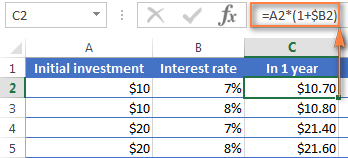As you remember, 1% is one part of a hundred, i.e. 0.01, so 7% is 0.07, and this is how percentages are actually stored in Excel. Keeping this in mind, you can verify the result returned by the formula by performing a simple calculation of 10*(1+0.07) or 10*1.07 and make sure that your balance after 1 year will be \$10.70 indeed.

And now, let's calculate the balance after 2 years. So, how much will your \$10 deposit be worth in two years' time at an annual interest rate of 7%? The answer is \$11.45 and you can get it by copying the same formula to column D.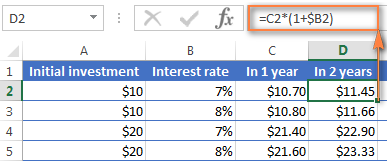To calculate how much money you will find in your bank account at the end of 3 years, simply copy the same formula to column E and you will get \$12.25.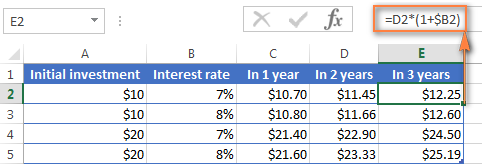Those of you who have some experience with Excel formulas have probably figured out that what the above formula actually does is multiplying the initial deposit of \$10 by 1.07 three times:

`=10*1.07*1.07*1.07=12.25043`

Round it to two decimal places and you will get the same number as you see in cell E2 in the screenshot above - \$12.25. Naturally, you can directly calculate the balance after 3 years using this formula:

`=A2*1.07*1.07*1.07`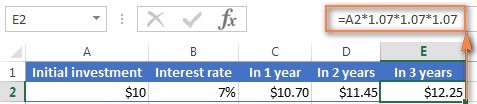#### Annual compound interest - formula 2

Another way to make an annual compound interest formula is to calculate the earned interest for each year and then add it to the initial deposit.

Assuming that your Initial deposit is in cell B1 and Annual interest rate in cell B2, the following formula works a treat:

`=B1 + B1 * \$B\$2`For the formula to work correctly, please mind the following details:

• Fix the reference to the Annual Interest Rate cell (B2 in our case) by adding the \$ sign, it should be an absolute column and absolute row, like \$B\$2.
• For Year 2 (B6) and all subsequent years, change the formula to:
Year 1 balance + Year 1 balance * Interest Rate

In this example, you'd enter the following formula in cell B6 and then copy it down to other rows, like demonstrated in the screenshot below:

`=B5 + B5 * \$B\$2`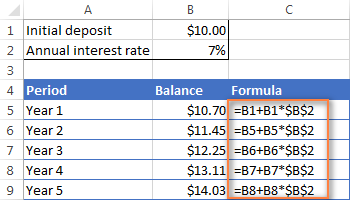To find out how much interest you actually earned with annual compounding, subtract the Initial deposit (B1) from Balance after 1 year (B5). This formula goes to C5:

`=B5-B1`

In C6, subtract Balance after 1 year from Balance after 2 years, and drag the formula down to other cells:

`=B6-B5`

You should see the earned interest growth like in the screenshot below.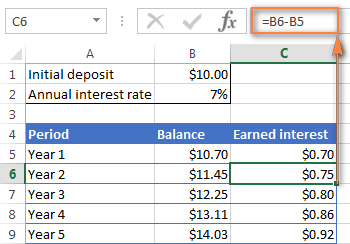The above examples do a good job illustrating the idea of compound interest, don't they? But none of the formulas is good enough to be called a universal compound interest formula for Excel. Firstly, because they do not let you specify a compounding frequency, and secondly, because you have to build an entire table rather than simply enter a certain duration and interest rate.

Well, let's take a step forward and create a universal compound interest formula for Excel that can calculate how much money you will earn with yearly, quarterly, monthly, weekly or daily compounding.

### General compound interest formula

When financial advisors analyze the impact of compound interest on an investment, they usually consider three factors that determine the future value of the investment (FV):

• PV - present value of the investment
• i - interest rate earned in each period
• n - number of periods

By knowing these components, you can use the following formula to get the future value of the investment with a certain compounded interest rate:

FV = PV * (1 + i)n

To illustrate the point better, here are a couple of quick examples.

#### Example 1: Monthly compound interest formula

Suppose, you invest \$2,000 at 8% interest rate compounded monthly and you want to know the value of your investment after 5 years.

First off, let's write down a list of components for your compound interest formula:

• PV = \$2,000
• i = 8% per year, compounded monthly (0.08/12= 006666667)
• n = 5 years x 12 months (5*12=60)

Input the above numbers in the formula, and you will get:

`= \$2,000 * (1 + 0.8/12)5x12 `

or

`= \$2,000 * 1.00666666760`

or

`= \$2,000 * 1.489845708 = \$2,979.69`

#### Example 2: Daily compound interest formula

I hope the monthly compound interest example is well understood, and now you can use the same approach for daily compounding. The initial investment, interest rate, duration and the formula are exactly the same as in the above example, only the compounding period is different:

• PV = \$2,000
• i = 8% per year, compounded daily (0.08/365 = 0.000219178)
• n = 5 years x 365 days (5*365 =1825)

Supply the above numbers into the compound interest formula, and you will get the following result:

`=\$2,000 * (1 + 0.000219178)1825 = \$2,983.52`

As you see, with daily compounding interest, the future value of the same investment is a bit higher than with monthly compounding. This is because the 8% interest rate adds interest to the principal amount each day rather than each month. As you can guess, the monthly compounding result will be higher than annual compounding.

All this is good, but what you really want is an Excel formula for compound interest, right? Just bear with me for a little longer, please. Now we are getting to the most interesting part - building your own powerful and versatile compound interest calculator in Excel.

### Compound interest formula in Excel (daily, weekly, monthly, yearly compounding)

Usually, there is more than one way to do something in Excel and a compound interest formula is not an exception :) Although Microsoft Excel provides no special function for calculating compound interest, you can use other functions to create your own compound interest calculator.

Let's start creating our Excel compound interest calculator with entering the basic factors that determine the future value of an investment in an Excel worksheet:

• initial investment (B3)
• annual interest rate (B4)
• number of compounding periods per year (B5)
• number of years (B6)

When done, your Excel sheet may look similar to this: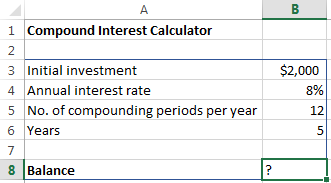All you need now is the compound interest formula to calculate the earned amount (Balance) based on the input values. The best news is that you don't have to re-invent the wheel. We will simply take the time-tested compound interest formula used by banking and other financial institutions and translate it into Excel's language.

Compound interest formula for Excel:

Initial investment * (1 + Annual interest rate / Compounding periods per year) ^ (Years * Compounding periods per year)

For the above source data, the formula takes this shape:

`=B3 * (1 + B4 /B5) ^ (B6 * B5)`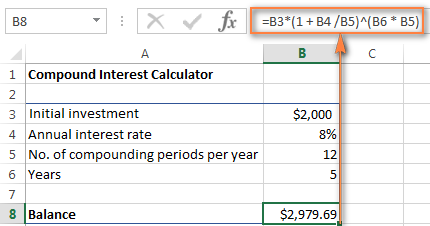The numbers look rather familiar? Yep, these are the same values and calculations that we've performed with a monthly compound interest formula, and the result proves that we did everything right!

If you want to know how much your investment will be worth at an 8% annual interest rate compounded quarterly, simply enter 4 in cell B5: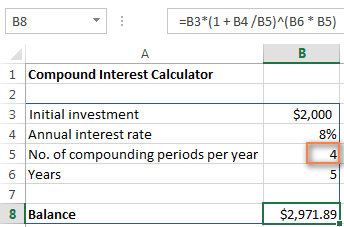To calculate the future value of your investment with semi-annual compounding, enter 2 as the Compounding periods per year value. For weekly interest rates, enter 52, this is how many weeks each year contains. If you are interested in daily compounding, enter 365, and so on.

To find the amount of earned interest, simply compute the different between the future value (balance) and the present value (initial investment). In our case, the formula in B9 is as simple as:

`=B8-B3`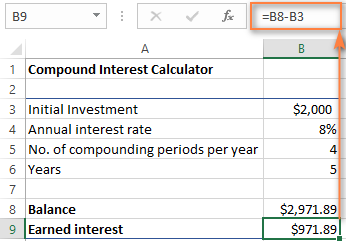As you see, we've created a truly universal compound interest calculator for Excel. Hopefully, now you have no regrets that you invested a few precious minutes in figuring out the tricky compound interest formula used by financial planners : )

### Advanced compound interest calculator for Excel

If for some reason you are not quite happy with the above approach, you can create your Excel compound interest calculator using the FV function that is available in all versions of Excel 2000 to 2019.

The FV function calculates the future value of an investment based on the input data similar to the ones we've discussed, though its syntax is a bit different:

FV(rate, nper, pmt, [pv], [type])

The detailed explanation of the arguments can be found in the Excel FV function tutorial.

In the meantime, let's build a FV formula using the same source data as in monthly compound interest example and see whether we get the same result.

As you may remember, we deposited \$2,000 for 5 years into a savings account at 8% annual interest rate compounded monthly, with no additional payments. So, our compound interest formula goes as follows:

`=FV(0.08/12, 5*12, ,-2000)`

If you need some explanation of the parameters, here you go:

• rate is 0.008/12 since you have the 8% annual interest rate compounded monthly.
• nper is 5*12, i.e. 5 years * 12 months
• pmt is left blank because we have no additional payments.
• pv is -2000 since it's an outflow and should be represented by a negative number.

Enter the above formula in an empty cell, and it will output \$2,979.69 as the result (which is perfectly inline with the result of the math calculation performed in the monthly compound interest example).

Naturally, nothing prevents you from replacing the values with cell references:

`=FV(B4/B5, B6*B5, , -B3)`

The screenshot below shows the future value of \$4,000 investment after 15 years at an annual interest rate of 7% compounded weekly: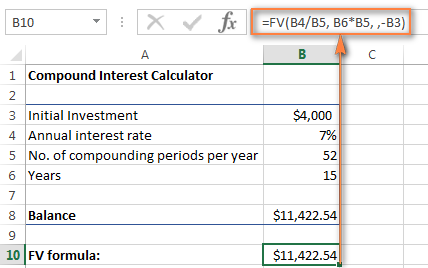To make your Excel compound interest calculator even more powerful, you can extend it with the Additional contributions option (additional payments) and modify the compound interest formula accordingly.

`=FV(B4/B5, B6*B5, -B8, -B3, B9)`

Where:

• B3 - principal investment
• B4 - annual interest rate
• B5 - the number of compounding periods per year
• B6 - the number of years to save
• B8 - additional contributions (optional)
• B9 - additional contributions type. Remember that you enter 1 if you deposit an additional amount at the beginning of the compounding period, 0 or omitted if additional payments are made at the end of the period.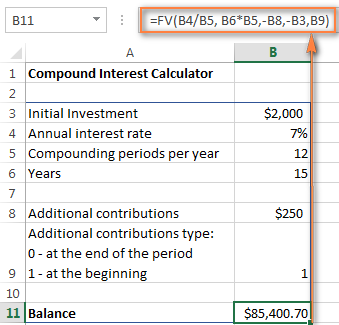If you are curious to try this advanced compound interest calculator for Excel to compute your savings, you can download it at the end of this post.

## Compound interest calculators online

If you prefer investing money rather than time in figuring out how to calculate compound interest in Excel, online compound interest calculators may come in handy. You can find plenty of them by entering something like "compound interest calculator" in your preferred search engine. In the meantime, let me quickly present a couple of my favorite ones.

#### Compound interest calculator by Bankrate

The key benefits of Bankrate compound interest calculator is ease-of-use and visual presentation of the results. This calculator lets you enter the savings inputs manually in boxes or by moving a slider. As you do this, the estimated total is displayed on top and immediately reflected in the graph below: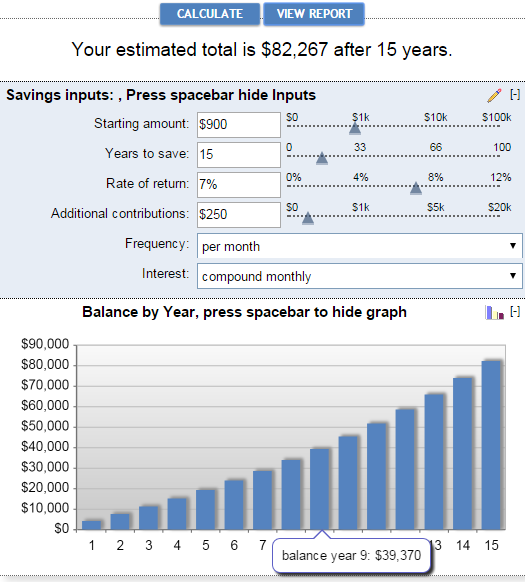Clicking the View Report button generates a "Summary Report" as well as "Savings Balance" that provides the detailed info on the amount of additional contributions, earned interest and balance for each year.

#### Compound interest calculator by Money-Zine

The online calculator from Money-Zine is much simpler compared to Bankrate's one. It asks you to specify only 3 values: the principal investment, interest rate and duration. As soon as you supply these numbers and click the Calculate button, it will show you all types of compound interest rate (daily, weekly, monthly, annual, etc.) as well as the future values with a corresponding compounding.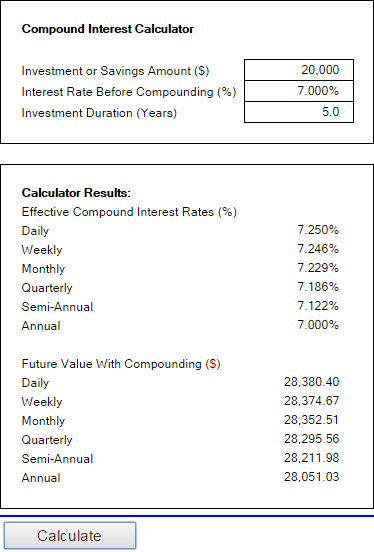#### Compound interest calculator by MoneySmart

This is a really nice online compound interest calculator run by Australian Securities and Investments Commission. It lets you input all relevant factors that determine the future value of your investment and outputs the result as a graph. By hovering over a certain bar in the graph, you can see the summary info for that particular year.This is how you calculate compound interest in Excel and outside it :) I hope at least one compound interest formula discussed in this article has proved helpful to you. Anyway, I thank you for reading and hope to see you on our blog next week!War in Ukraine. Here's what Ablebits is doing to make sure our team and projects are safe.